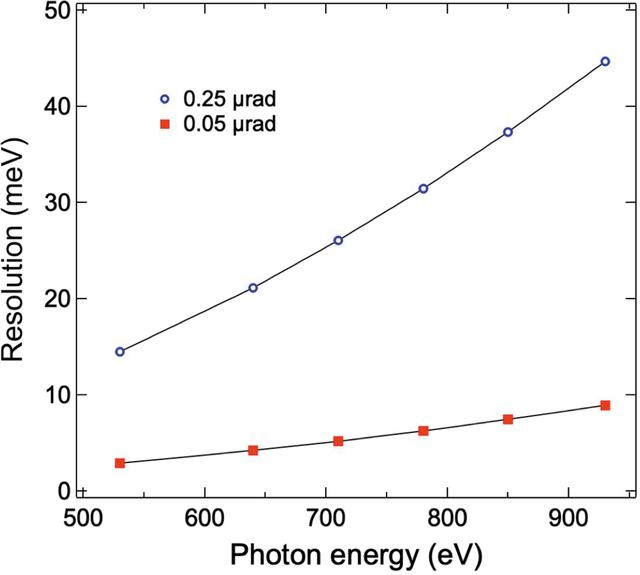disable zoom     view article Figure 10 Estimated resolution contributed from the slope error of the grating as a function of photon energy. Red squares and blue circles represent the combined resolution of the AGM and AGS gratings contributed from slope errors of 0.05 and 0.25 µrad r.m.s., respectively. The slope error contributionis calculated through the grating equation, i.e., where λ and m are the wavelength and grating diffraction order, respectively, α and β are the grating incidence and diffraction angles from the surface normal, respectively, Δϕ is the grating slope error and d is the grating period. Black solid lines serve as a guide to the eye.JOURNAL OFSYNCHROTRONRADIATION
ISSN: 1600-5775
Volume 28| Part 3| May 2021| Pages 977-986